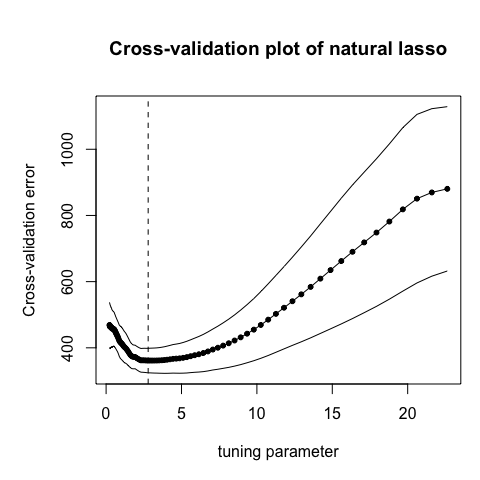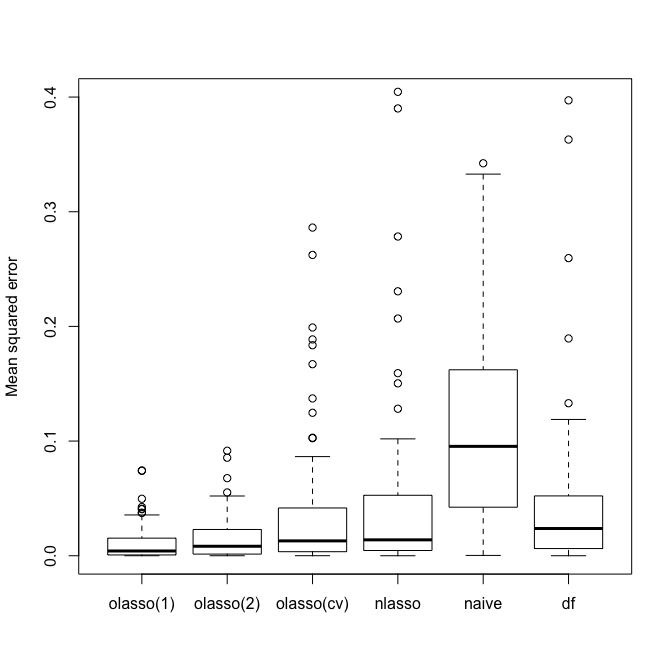# Using the natural package

#### 2018-01-15

The natural package contains the implementations of two methods that estimate the error variance of a high-dimensional linear model, namely, the natural lasso and the organic lasso. The details of the methods can be found in Yu, Bien (2017) Estimating the error variance in a high-dimensional linear model. In particular, given a data matrix $$X \in \mathbb{R}^{n \times p}$$, with each row an observation of $$p$$ features, and a vector of response $$\mathbf{y} \in \mathbb{R}^n$$, this package implements two penalized maximum likelihood-based approaches for jointly estimating $$\beta$$ and $$\sigma^2$$ in a linear model $y = X \beta + \varepsilon, \quad \varepsilon \sim N(0, \sigma^2).$ This document serves as an introduction of using the package.

## Data simulation

To reproduce the simulation study in the paper, the package also contains a function to generate random samples from a linear model with user-specified model parameters. In particular, make_sparse_model generates a sparse linear model as above, with $$X \sim N(0, \Sigma)$$ such that $$\Sigma_{jj} = 1$$ and $$\Sigma_{ij} = \rho$$. The value of columnwise correlation $$\rho$$ is set by the function argument rho. To generate $$\beta$$, we set the number of nonzero elements to be $$\lceil n^\alpha \rceil$$, where $$\alpha$$ is set by the argument alpha, and each nonzero element is drawn from a Laplace distribution of rate $$1$$. For a given signal-to-noise ratio, as specified by snr, we have error variance $$\sigma^2 = \beta^T \Sigma \beta / snr$$.

library(natural)
set.seed(123)
nsim <- 100
sim <- make_sparse_model(n = 50, p = 300, alpha = 0.6, rho = 0.6, snr = 2, nsim = nsim)

## Estimating $$\sigma$$ with natural lasso

The main functions implementing natural lasso are nlasso_path and nlasso_cv. nlasso_path computes the natural lasso estimates of the error variance along a path of tuning parameters, and nlasso_cv selects the tuning parameter using K-fold cross-validation.

### Computing natural lasso along a path of tuning parameters

nlasso_path takes the design matrix and the response . It also requires a path of tuning parameters $$\lambda$$, and the function outputs the following three estimates:

sig_obj_path $\hat{\sigma} ^2(\lambda) = \frac{1}{n}||y - X \hat{\beta}_\lambda||_2^2 + 2 \lambda ||\hat\beta_\lambda||_1,$ sig_naive_path $\hat{\sigma} ^2_{naive}(\lambda) = \frac{1}{n}||y - X \hat{\beta}_\lambda||_2^2,$ and sig_df_path (Reid, et, al 2016) $\hat{\sigma}^2_{df}(\lambda) = \frac{1}{n - \hat{s}_\lambda}||y - X \hat{\beta}_\lambda||_2^2,$ where $\hat{\beta}_\lambda = \arg\min \frac{1}{n} ||y - X \beta||_2^2 + 2\lambda ||\beta||_1$ is the lasso solution with tuning parameter $$\lambda$$, and $$\hat{s}_\lambda$$ is the degree of freedom of the lasso fit.

The tuning parameter path can be specified via argument lambda. If not provided, the algorithm will automatically generate a path of lambda of length nlam. The output is a S3 object, which can be printed or plotted.

nl <- nlasso_path(x = sim$x, y = sim$y[, 1])
#plot(nl)
#print(nl)

### Selecting the tuning parameter of natural lasso using cross-validation

The function nlasso_cv implements a $$K$$-fold cross-validation procedure to select the best tuning parameter value. The value of $$K$$ can be specified by the argument nfold. The following code does the cross-validation, plots the estimate of prediction error on the test set, and selects the best tuning parameter.

nl_cv <- nlasso_cv(x = sim$x, y = sim$y[, 1])
plot(nl_cv)The return of nlasso_cv is a list of objects. See ?nlasso_cv for more details. In particular, sig_obj, sig_naive, and sig_df are the cross-validated estimates.

### Computing natural lasso with glmnet output

The function nlasso_path calls glmnet internally to solve lasso problems. In many use cases, one might have already called glmnet (and/or cv.glmnet) before calling nlasso_path and/or nlasso_cv. To avoid redundant computation, one can pass the output from glmnet into nlasso_path using the argument glmnet_output. By doing so, arguments like lambda, nlam, flmin, etc, will be ignored, and the function will compute estimates of $$\sigma$$ from glmnet_output directly. It is suggested that glmnet_output should be from glmnet call with argument standardize = TRUE (which is by default) to align with what nlasso_path is doing internally when glmnet_output = NULL (by default).

library(glmnet)
## Loading required package: Matrix
## Loading required package: foreach
## Loaded glmnet 2.0-13
g_o <- glmnet(x = sim$x, y = sim$y[, 1], lambda = nl$lambda) nl2 <- nlasso_path(x = sim$x, y = sim$y[, 1], glmnet_output = g_o) Similarly, one can pass the output from cv.glmnet into nlasso_cv with argument glmnet_output. g_o_cv <- cv.glmnet(x = sim$x, y = sim$y[, 1]) nl_cv2 <- nlasso_cv(x = sim$x, y = sim$y[, 1], glmnet_output = g_o_cv) ## Estimating $$\sigma$$ with organic lasso Organic lasso is a companion method to the natural lasso. The main novelty is that the choice of tuning parameter is pivotal, in that it does not depend on any unknown parameter. The organic lasso estimate of the error variance is defined as $\tilde{\sigma}_\lambda^2 = \min_{\beta} \frac{1}{n} ||y - X\beta||_2^2 + 2 \lambda ||\beta||_1^2.$ The main functions implementing organic lasso are olasso_path, olasso_cv, and olasso. In particular, olasso_path computes the organic lasso estimates of the error variance along a path of tuning parameters, and olasso_cv selects the optimal tuning parameter using a $$K$$-fold cross-validation procedure. The usages are the same as nlasso_path and nlasso_cv. Please see ?olasso_cv and ?olasso_path for more details. The function olasso computes the organic lasso estimate of $$\sigma$$ corresponding to two pre-specified values of tuning parameters. In particular, the function outputs the organic lasso estimates with $$\lambda_1 = \frac{\log p}{n}$$, and $$\lambda_2$$, which is a Monte-Carlo estimate of the quantity $$n^{-2}||X^T e||_\infty^2$$, where $$e$$ is an n-dimensional vector of independent standard normals. We show in the following example that both of them give close estimates of the true error variance. For completeness of the comparison, we also include the outputs of olasso_cv and nlasso_cv. err_o_mat <- matrix(NA, nrow = nsim, ncol = 6) colnames(err_o_mat) <- c("olasso(1)", "olasso(2)", "olasso(cv)", "nlasso", "naive", "df") for(i in seq(nsim)){ cur_ol <- olasso(x = sim$x, y = sim$y[, i]) err_o_mat[i, 1] <- (cur_ol$sig_obj_1 / sim$sigma - 1)^2 err_o_mat[i, 2] <- (cur_ol$sig_obj_2 / sim$sigma - 1)^2 cur_ol_cv <- olasso_cv(x = sim$x, y = sim$y[, i]) err_o_mat[i, 3] <- (cur_ol_cv$sig_obj / sim$sigma - 1)^2 cur_nl_cv <- nlasso_cv(x = sim$x, y = sim$y[, i]) err_o_mat[i, 4] <- (cur_nl_cv$sig_obj / sim$sigma - 1)^2 err_o_mat[i, 5] <- (cur_nl_cv$sig_naive / sim$sigma - 1)^2 err_o_mat[i, 6] <- (cur_nl_cv$sig_df / sim\$sigma - 1)^2
}
boxplot(err_o_mat, ylim = c(0, 0.4), ylab = "Mean squared error")